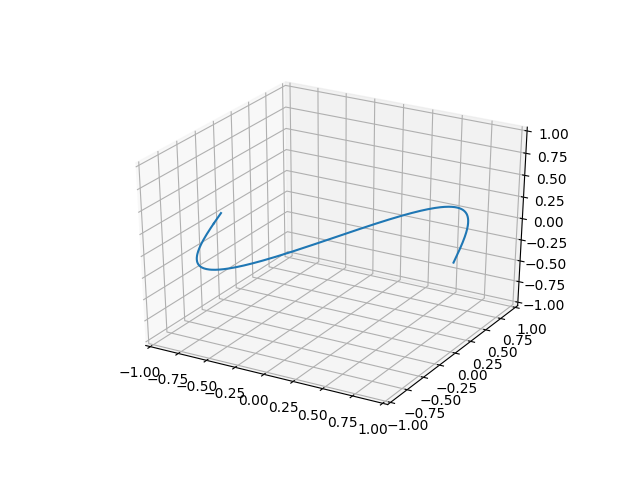# Tutorial¶

In this tutorial, we will show the use of NodeFinder with a simple example. We will find the nodal line given by the following function:

```In : import numpy as np

In : def gap_function(pos):
...:     x, y, z = pos
...:     return np.sqrt((y - np.sin(np.pi * x))**2 + z**2)
...:
```

## Identify¶

The second step in the process is to identify the nodal features from the point clouds calculated in the search step. For this purpose, the `identify` submodule is used.

This process works by first clustering the points into connected components. Next, the dimension of each cluster is determined. Finally, the shape of the object is determined.

```In : identify_result = nf.identify.run(search_result)

In : print(identify_result)
IdentificationResultContainer(coordinate_system=CoordinateSystem(limits=array([[-1,  1],
[-1,  1],
[-1,  1]]), periodic=True), feature_size=0.1, results=[IdentificationResult(dimension=1, shape=NodalLine(graph=<1468 nodes, 1468 edges>, degree_count=Counter(), shape_name='CLOSED LOOP'), positions=<1533 values>)])

In : nf.identify.plot.result(identify_result);
```